# Trigonometry

### Term 1 starting in week 4 :: Estimated time: 3 weeks

• Explore ratio in similar right-angled triangles
• Work fluently with the hypotenuse, opposite and adjacent sides
• Use the tangent ratio to find missing side lengths
• Use the sine and cosine ratio to find missing side lengths
• Use sine, cosine and tangent to find missing side lengths
• Use sine, cosine and tangent to find missing angles
• Calculate sides in right-angled triangles using Pythagoras' Theorem (review)
• Select the appropriate method to solve right-angled triangle problems
• Work with key angles in right-angled triangles

For higher-attaining pupils:

• Use trigonometry in 3-D shapes Use the formula ½ ab sin C to find the area of a triangle
• Understand and use the sine rule to find missing lengths
• Understand and use the sine rule to find missing angles
• Understand and use the cosine rule to find missing lengths
• Understand and use the cosine rule to find missing angles
• Choosing and using the sine and cosine rules

This page should remember your ticks from one visit to the next for a period of time. It does this by using Local Storage so the information is saved only on the computer you are working on right now.

## Lesson Starters

Here are some suggestions for whole-class, projectable resources which can be used at the beginnings of each lesson in this block.

### 1st Lesson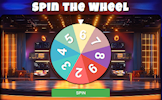#### One Torch Tunnel

Work out the least amount of time for four people to walk through a tunnel?

### 2nd Lesson#### Moon Lengths

Estimate the distances shown on this photograph of the moon's surface.

### 3rd Lesson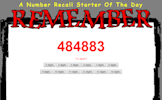#### Number Recall

Can you improve your ability to remember telephone numbers?

### 4th Lesson#### Vowelless

Vowels have been taken out of mathematical words. Can you recognise them?

### 5th Lesson#### Pears Make Squares

Find three numbers such that each pair of numbers adds up to a square number.

### 6th Lesson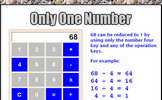#### Only One Number

Find other numbers that can be changed to 1 on a calculator using only the 4 key and any operation.

### 7th Lesson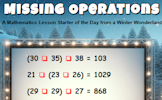#### Missing Operations

Each red box represents a missing operation (+, -, x or ÷). Can you work out what they are?

### 8th Lesson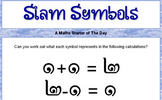#### Siam Symbols

Can you work out what each of the strange symbols represents in these calculations?

### 9th Lesson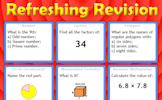#### Refreshing Revision

It is called Refreshing Revision because every time you refresh the page you get different revision questions.

Some of the Starters above are to reinforce concepts learnt, others are to introduce new ideas while others are on unrelated topics designed for retrieval practice or and opportunity to develop problem-solving skills.

White Rose ResourcesEnd of block assessments provide a quick progress check at the end of each block of learning to make sure students have understood the content covered. This Scheme of Learning was produced by White Rose Maths and is used here with permission granted on 30th June 2021.For All: